# Martin Orr's Blog

## The Faltings height and normed modules

Posted by Martin Orr on Saturday, 31 December 2011 at 15:31

In this post I shall give the definition of the Faltings height of an abelian variety over any number field. Last time we did this over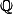only, and we used two properties of: the integers are a PID and there is only one archimedean place. To do things more generally, we will introduce the technology of normed modules and their degrees.

### Normed modules

Let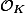be the ring of integers of a number field. We define a normed-module to be a projective-moduleof finite rank together with a norm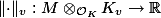for each archimedean placeof.

(A norm is a functionsatisfyingif,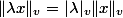if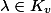, and the triangle inequality.)

Our primary example of a normed module is the canonical module of the Néron model of an abelian variety: letbe an abelian variety of dimensionandits Néron model. Thenis a projective-module of rank 1, and we get a norm onas follows: ifthen we can extenduniquely to a global canonical formon. We define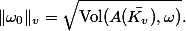We now define the degree of a normed module of rank 1, which gives us the Faltings height of an abelian variety. (It seems a bit odd to me to call this a degree, because I expect a degree to be an integer, but I believe that the justification comes from Arakelov intersection theory.)

If, then we simply choose a generatorof(unique up to sign) and defineto be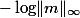.

Over other number fields, there are two problems with this definition. Firstly there may be more than one archimedean place, so which one do we choose? We simply take a sum over all of them.

More seriously, the modulemight not be principal, so there is no generator. To get around this, we choose anyat all, and add on a term that measures how faris from being a generator. Specifically,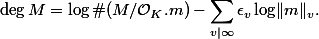Here(which is 1 or 2).

Note that by the Chinese remainder theorem, we can write the first term ofas a sum over the finite places of:The expression foris independent of the choice ofby the product formula for absolute values.

### Faltings heights

We define the (unstable) Faltings height of an abelian varietyusing the degree of the canonical module of its Néron model:The factorreduces the dependence on the base field, but does not eliminate it. Specifically, ifis a normed-module, then we haveHowever the Néron model of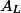need not be the base change of the Néron model of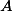, so that. For example an elliptic curve with additive reduction may have good reduction after a finite extension of the base field.

We say that an abelian varietyhas semistable reduction if every reduction of its Néron model is a semiabelian variety, that is an extension of an abelian variety by a torus. In the case of an elliptic curve, this is saying that it has good or multiplicative reduction everywhere.

We have the following two facts:

Fact 1. Any abelian varietyhas semistable reduction over some finite extension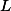of.

Fact 2. Ifhas semistable reduction, then the identity component of its Néron model is unchanged by finite extensions of the base field.

So we define the stable Faltings height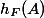to be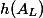for some finite extensionsuch thathas semistable reduction. By fact 2 it does not matter whichwe pick, and the stable Faltings height is independent of the base field, so it is often more convenient than the unstable Faltings height.

1. The Chowla-Selberg formula From Martin's Blog

The Chowla-Selberg formula is an equation which expresses the periods of CM elliptic curves in terms of values of the gamma function at rational arguments. Colmez conjectured a generalisation of the Chowla-Selberg formula to higher-dimensional CM ...

1. Barinder Banwait said on Friday, 03 February 2012 at 20:54 :

Letbe a curve over a number field, andits Jacobian variety. There are various notions of the height of, and I'll let you choose your favourite one. Is there any link between the height of, and the Faltings height of?

2. Barinder Banwait said on Saturday, 04 February 2012 at 02:00 :

Allow me a moment of shameless self-advertising to the readership of Martin's blog.

Barinder Banwait, frequent commentator on Martin's blog, has setup his own blog at bbanwait.wordpress.com. He will be discussing Mazur's Isogeny Theorem, with a view towards the Uniform Boundedness Theorem. But he may not get there. Why not check out what the fuss is about?